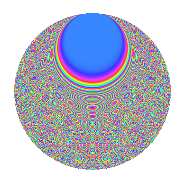# Properties

 Label 2541.2.uLevel 2541 Weight 2 Character orbit u Rep. character $$\chi_{2541}(251,\cdot)$$ Character field $$\Q(\zeta_{10})$$ Dimension 1088 Sturm bound 704

# Related objects

## Defining parameters

 Level: $$N$$ = $$2541 = 3 \cdot 7 \cdot 11^{2}$$ Weight: $$k$$ = $$2$$ Character orbit: $$[\chi]$$ = 2541.u (of order $$10$$ and degree $$4$$) Character conductor: $$\operatorname{cond}(\chi)$$ = $$231$$ Character field: $$\Q(\zeta_{10})$$ Sturm bound: $$704$$

## Dimensions

The following table gives the dimensions of various subspaces of $$M_{2}(2541, [\chi])$$.

Total New Old
Modular forms 1504 1216 288
Cusp forms 1312 1088 224
Eisenstein series 192 128 64

## Trace form

 $$1088q + 268q^{4} + 2q^{7} + 12q^{9} + O(q^{10})$$ $$1088q + 268q^{4} + 2q^{7} + 12q^{9} - 180q^{16} - 2q^{18} - 184q^{25} - 8q^{28} - 24q^{30} + 54q^{36} - 12q^{37} + 66q^{39} + 50q^{42} - 36q^{46} - 30q^{49} - 38q^{51} + 86q^{57} + 136q^{58} - 8q^{60} - 44q^{63} + 204q^{64} - 224q^{67} - 142q^{70} - 86q^{72} + 20q^{78} + 156q^{79} - 76q^{81} + 30q^{84} - 8q^{85} - 86q^{91} - 48q^{93} + O(q^{100})$$

## Decomposition of $$S_{2}^{\mathrm{new}}(2541, [\chi])$$ into newform subspaces

The newforms in this space have not yet been added to the LMFDB.

## Decomposition of $$S_{2}^{\mathrm{old}}(2541, [\chi])$$ into lower level spaces

$$S_{2}^{\mathrm{old}}(2541, [\chi]) \cong$$ $$S_{2}^{\mathrm{new}}(231, [\chi])$$$$^{\oplus 2}$$

## Hecke Characteristic Polynomials

There are no characteristic polynomials of Hecke operators in the database